• 主要介绍了Java实现冒泡排序双向冒泡排序算法的代码示例,值得一提的是所谓的双向冒泡排序并不比普通的冒泡排序效率来得高,注意相应的时间复杂度,需要的朋友可以参考下
• 题目链接
题目链接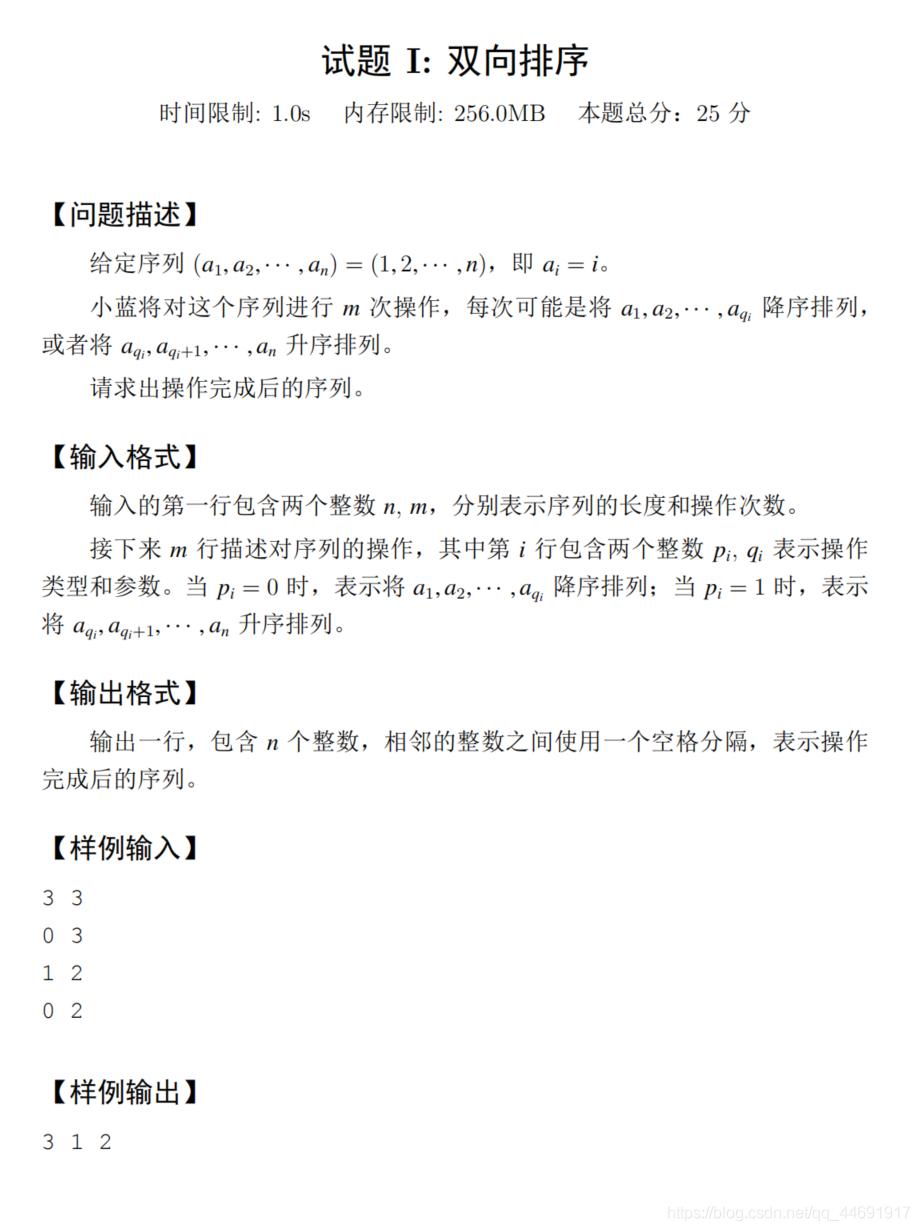题解：
比赛时就直接写了一个暴力sort交上，能骗一点分是一点 昨晚看了acwing的讲解，现在结合我的思路更新正解 题目中设计两个操作，一个是选定前x个数，使其降序，另一个是选定后y个数，使其升序 问最后操作完，输出序列 题目就两个操作，我们考虑多种情况： 1.连续出现多个操作一。图中蓝色区域为操作1，如果连续出现多个操作1，虽然区间有长有短，但效果都是将区间内降序，我们不难发现最后有效果的是最长的区间部分（即图中第三个蓝区间），因为这个操作排完序后，之后连续的操作1都不会其效果（因为已经排好序） 说的有些啰嗦，总结：连续操作1取最长区间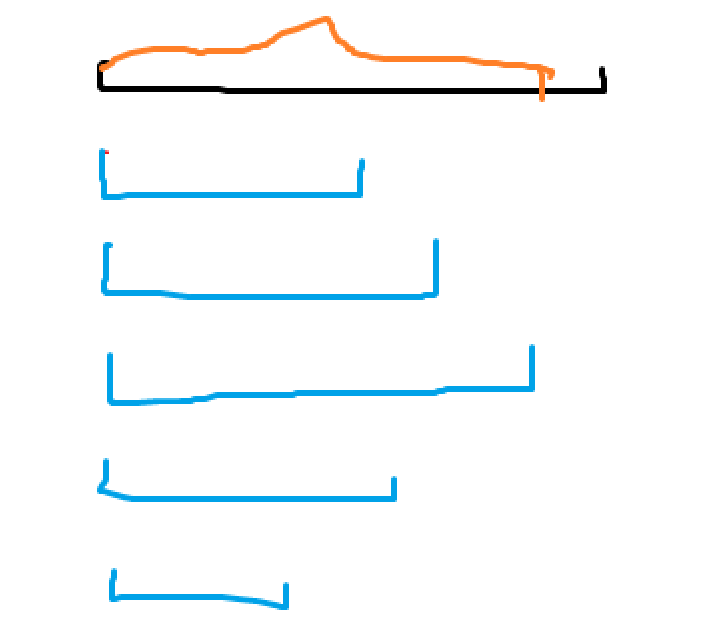2.连续的操作2 同理：连续的操作2，我们也取最长部分 情况1和情况2使得最终的操作是1和2更替进行，（因为如果有连续就取最长）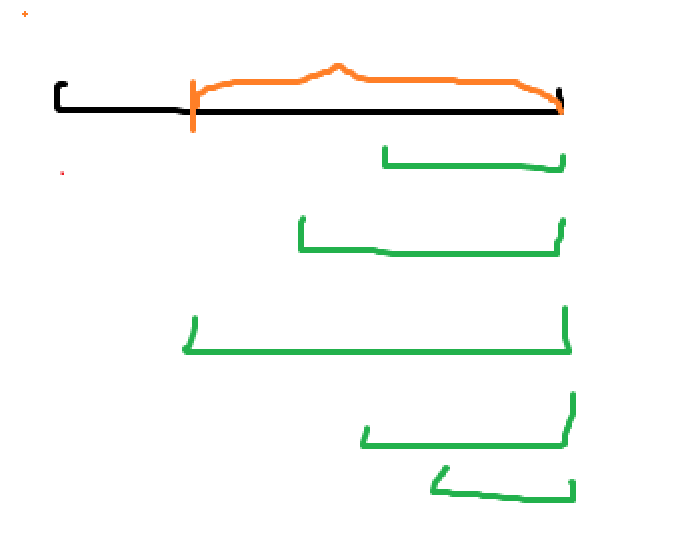3.操作1与操作2交替 图中蓝色部分为操作1，绿色部分为操作2， 首先我们要知道序列一开始是升序的（即黑色区间），所以F中的数<G<H 第一个操作肯定是操作1，因为操作2没影响 操作1为降序，B>A，而绿区间为升序，我们要注意H区间的数是大于F和G的，我们操作1只对区间A，B进行了修改，所以H>A和B，那么操作2对C和D区间（即G和H）进行修改时，D区间保持不变，（因为D区间本来就是升序，为啥要变）但是C区间目前是降序，所以要变成升序。 这样看来，改变的只有 接下来应该是操作1了，如果接下来的操作1比之前的操作1的区间长度长，那之前的操作1的区间就可以省略，因为后来的操作完全将之前的覆盖了，如果把之前的操作1省略掉，就会出现两个操作2相邻，为了保证两个操作交替进行，所以上一个操作1和操作2都会消失（看下面第二个图中被矩形选中的部分为省略） 因为我们可以得到，操作1和2交替进行，且相比于上一次操作，本次操作的区间长度越来越短，因此相交部分也越来越短，直到两者不再相交，到这时修改将不再起作用，每次操作其实反转的也只有相交部分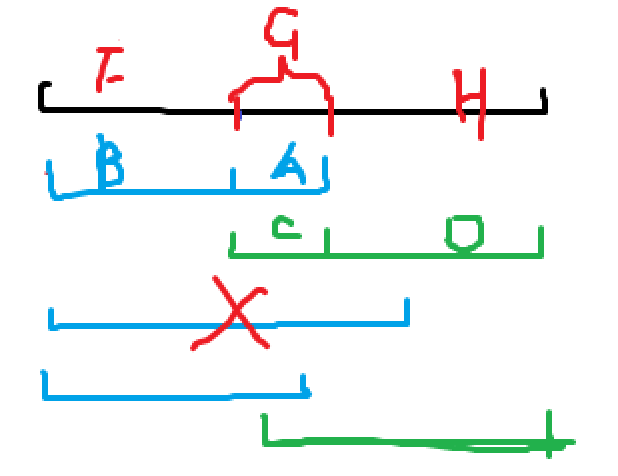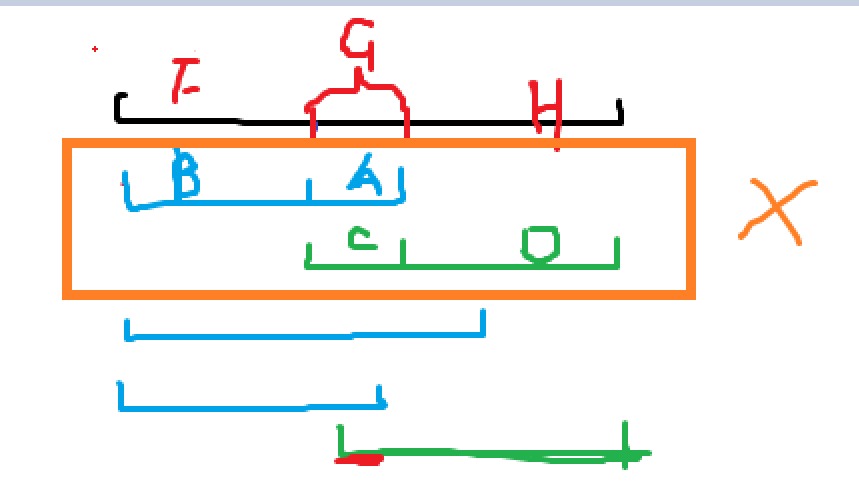我们用这个图来总结一下：红色线段部分修改，蓝色线段保持上一次状态 我们设红色线段的两端分别是x和y，因为我们每次要做的就是反转区间[x,y]，且x和y是不断向内靠近的 反转的操作可以用平衡树或者线段树，但是没必要 因为我们说了相交区间越来越小，当执行操作1时，本质是y向内靠，当执行操作2时，本质是x向内靠， 当x和y向内靠时，经过的点在后续不会发生改变，那我们就直接给他赋值即可，我们用一个变量K，K一开始为n，第一个操纵为1，我们要将y移动到第一个红线的右端（下图），移动过程中给非红线的部分一次赋值，并k–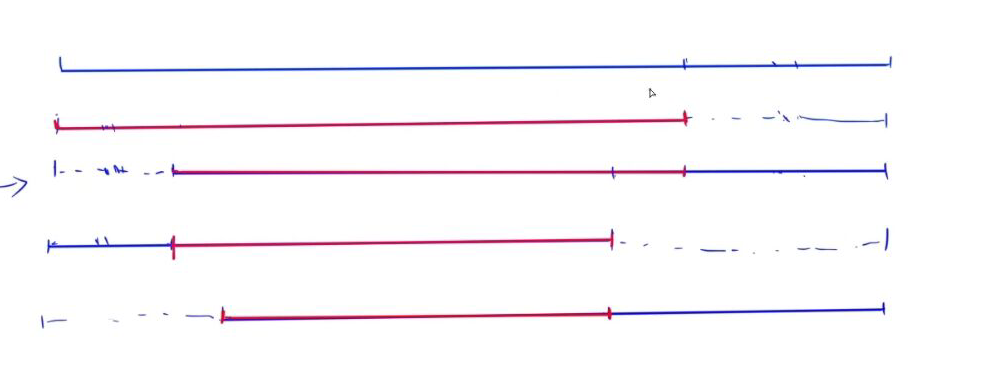最终实现的效果如下: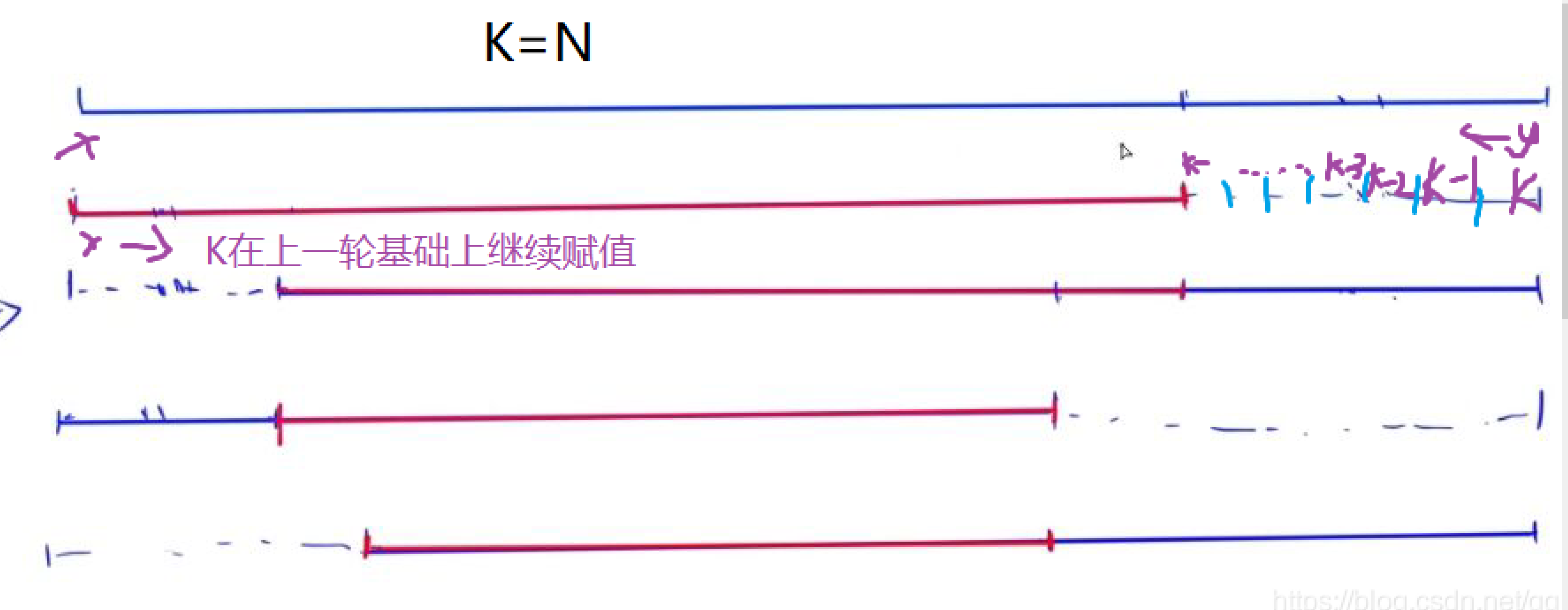这就实现了反转的操作 我讲的不是很清楚，有什么问题可以评论区里问
代码：
好题，代码为yxc写的，这个代码逻辑比我写的清晰 代码我有详细注释
#include <iostream>
#include <cstring>
#include <algorithm>

#define x first
#define y second

using namespace std;

typedef pair<int, int> PII;

const int N = 100010;

int n, m;
PII stk[N];
int ans[N];

int main()
{
scanf("%d%d", &n, &m);
int top = 0;
while (m -- )
{
int p, q;
scanf("%d%d", &p, &q);
if (!p)//操作1
{
while (top && stk[top].x == 0)
q = max(q, stk[top -- ].y);//出现连续的操作1，我们取最大
while (top >= 2 && stk[top - 1].y <= q)
//如果当前的操作1比上一次的操作1范围大，则将上一次操作1和操作2删除
top -= 2;
stk[ ++ top] = {0, q};//存本次最佳操作
}
else if (top)//操作2 &&且操作1已经进行过（操作二第一个用没效果）
{
while (top && stk[top].x == 1) q = min(q, stk[top -- ].y);
while (top >= 2 && stk[top - 1].y >= q) top -= 2;
stk[ ++ top] = {1, q};
}
}
int k = n, l = 1, r = n;
for (int i = 1; i <= top; i ++ )
{
if (stk[i].x == 0)//如果是操作1
while (r > stk[i].y && l <= r) ans[r -- ] = k -- ;//移动r值 ，并赋值
else
while (l < stk[i].y && l <= r) ans[l ++ ] = k -- ;
if (l > r) break;
}
if (top % 2)
while (l <= r) ans[l ++ ] = k -- ;
else
while (l <= r) ans[r -- ] = k -- ;

for (int i = 1; i <= n; i ++ )
printf("%d ", ans[i]);
return 0;
}

展开全文思维题
• 2021 第十二届蓝桥杯 双向排序 题解 思路 快排的时间复杂度是O(n*(n logn ))，考虑到可能会超时，所以比赛的时候想用文艺平衡


2021 第十二届蓝桥杯 双向排序 题解 栈+文艺平衡树
题目描述思路实现方法代码只用栈只用栈的代码

题目描述
原题链接:https://www.lanqiao.cn/problems/1458/learning/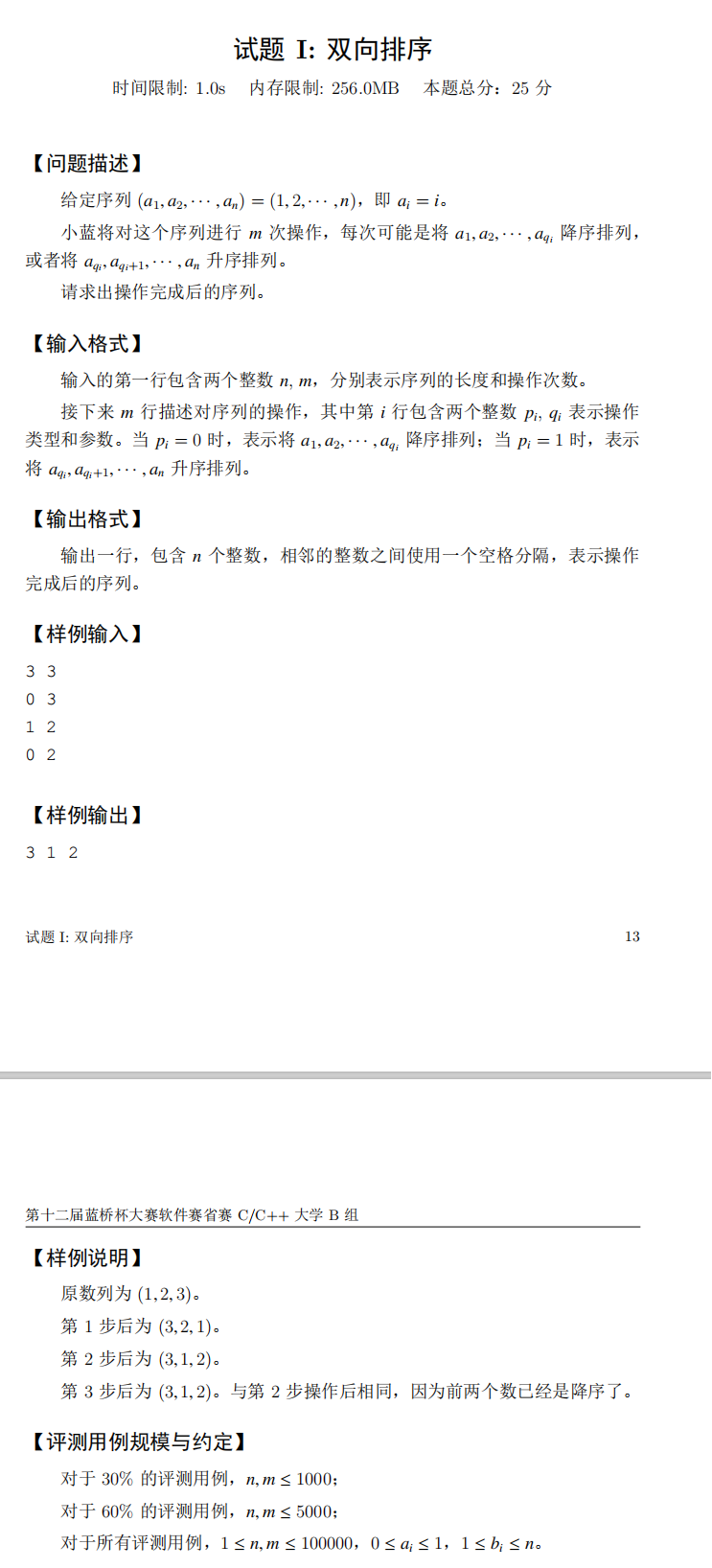思路
快排的时间复杂度是O(n*(n logn ))，考虑到可能会超时，所以比赛的时候想用文艺平衡树这种单次操作只需要最多O(logn)就能翻转任意区间的神奇数据结构，整体的时间复杂度一下子就降到了优秀的O(n logn)。
实现方法
本篇不详细介绍文艺平衡树，若您需要这项前置技能，那么强烈为您推荐洛谷模板题
如何操作？这里有一个难点，题目的要求是排序，而文艺平衡树的区间翻转是不能保证有序的，所以每次翻转区间一定是在一个有序的区间内部进行操作，才能保证答案的正确。
那这一点又如何保证呢？维护一个降序栈，使得每次翻转区间都在上一个翻转后的区间内部进行。 如果有两个连续的指令都是让我们排降序，比如0 3和0 5，那么0 3这条指令就不需要进行操作，因为只进行0 5这步操作 和 进行两步操作是一样的（需要稍微脑补一下）。 {1 2 3 4 5 6 7} 0 3 {3 2 1 4 5 6 7} 0 5 {5 4 3 2 1 6 7}
所以，连续的0类指令，只需要取最大的那个，连续的1类指令，只需要取最小的那个，于是最后我们就得到了一堆0类1类交替的指令，这一点很重要，我们需要维护0类1类指令的严格交替！观察一下下面的两个操作。 {1 2 3 4 5 6 7} 0 5 {5 4 3 2 1 6 7} 1 4 {5 4 3 1 2 6 7} 0 5的意义就是将[1,5]这个区间进行降序操作，而一开始整个区间[1,7]都是升序的,我们要让[1,5]降序，那就只需要取[1,7]和[1,5]的交集，然后翻转这个交集，显然这个交集就是[1,5]，翻转[1,5]，于是我们得到了一个降序的[1,5]区间，1 4的意义就是将[4,7]这个区间进行升序操作，而最右边的区间[6,7]已经是升序的了，并且里面所有的数字都比它们左边所有的数字都要大，所以[6,7]区间的数字并不会变化，我们只需要接着取交集，取[1,5]和[4,7]的交集，得到一个[4,5]的区间，翻转[4,5]就是我们最终的答案。
于是，你可能跟我一样，发现了华点，要是上面两个操作之后，突然出现一个0 7的指令怎么办？按照之前的说法，我们不能通过取 [1,7]和[4,5]的交集翻转，显然答案是错误的。
所以不是连续的0操作最大就行了，而是要让后面比较大的0操作，覆盖掉之前所有比它小的操作，其间的1和0操作都会因为这个比较大的0操作而变得无效。
考虑维护两个栈，或者用数组模拟写在一个栈里也行，0操作严格降序，1操作严格升序，并且一定要让0和1交替出现，最差的情况会长这样：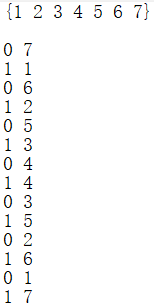这个栈维护完了之后就直接按照之前的方法一直循环取交集翻转就可以了，并且这个交集的长度只有1的时候就可以直接跳出了，后面的操作都没有意义。
本人Java C组，学了很多算法今天终于能用上了 很开心，最后一分钟的时候把那个栈维护出来了，非常兴奋交了上去。结果比赛结束后发现没有改类名！！！！铁定0分了，还不如直接sort呢 真是悲伤，java组的同胞们一定要引以为戒，仔细检查类名和包！
代码
import java.io.BufferedReader;
import java.io.BufferedWriter;
import java.io.IOException;
import java.io.OutputStreamWriter;
import java.io.PrintWriter;
import java.io.StreamTokenizer;

public class Main
{
static int[]val,f,siz;//val-值,f-当前节点的父亲,siz-当前节点的大小
static boolean[]lazy;//当前节点的 翻转懒标记
static int[][]ch;//ch[i]-i节点的左儿子,ch[i]-i节点的右儿子
static int len,n,root;//len用来初始化splay树,n-输入的n,root-splay树的根节点
static int init(int be,int ed,int fa)
{
/* 模访线段树的初始化方法
* 初始化splay
*/
if(be>ed)return 0;
int a=len++;
f[a]=fa;
int mid=(be+ed)>>1;
int l=init(be,mid-1,a);
val[a]=mid;
int r=init(mid+1,ed,a);
ch[a]=l;
ch[a]=r;
update(a);
return a;
}
static void pushdown(int a){
/*
* 向下传递懒标记
*/
if(lazy[a]){
int stack=ch[a];
ch[a]=ch[a];
ch[a]=stack;
lazy[ch[a]]^=true;
lazy[ch[a]]^=true;
lazy[a]=false;
}
}
static void connect(int a,int fa,int s){
if(fa!=0)ch[fa][s]=a;
if(a!=0)f[a]=fa;
}
static void update(int a){
siz[a]=siz[ch[a]]+siz[ch[a]]+1;
}
static void rotate(int a){
int fa=f[a],ffa=f[fa];
int s=ch[fa]==a?0:1;
connect(ch[a][1^s],fa,s);
connect(a,ffa,ch[ffa]==fa?0:1);
connect(fa,a,1^s);
update(fa);
update(a);
}
static int find(int s){
int a=root;
while(true){
pushdown(a);
if(siz[ch[a]]>=s)a=ch[a];
else {
s-=siz[ch[a]]+1;
if(s==0)return a;
a=ch[a];
}
}
}
static void splay(int a,int to){
while(f[a]!=to){
int fa=f[a];
if(f[fa]!=to)rotate((ch[fa]==a)==(ch[f[fa]]==fa)?a:fa);
rotate(a);
}
if(to==0)root=a;
}
static void re(int a,int b)
{
/*
* 翻转区间[a,b]
*/
splay(find(a),0);
splay(find(b+2),root);
lazy[ch[ch[root]]]^=true;
}
static boolean flag=false;
static void dfs(int a)
{
/*
* 最后dfs中序遍历输出
*/
pushdown(a);
if(ch[a]!=0)dfs(ch[a]);
if(val[a]>=1&&val[a]<=n){
if(flag)out.print(" ");
else flag=true;
out.print(val[a]);
}
if(ch[a]!=0)dfs(ch[a]);
}
public static void main(String[]args) throws IOException{
n=in();
ch=new int[n+3];
val=new int[n+3];
f=new int[n+3];
siz=new int[n+3];
lazy=new boolean[n+3];
len=1;
int m=in();
root=init(0,n+1,0);
int[]stack=new int[m];
int cnt=0;
while(m-->0){
int op=in();
int mid=in();
{//维护栈
if(op==0){
if(cnt%2!=op)//如果要放入的指令与栈中最后一个指令类型相同
{
if(cnt-1>=0&&stack[cnt-1]<=mid)cnt--;//则进行第一轮比较，如果当前更大则弹出最后一个
else continue;//否则直接舍去当前指令，进入下一层循环
}
while(cnt-2>=0&&stack[cnt-2]<=mid) cnt-=2;//循环弹出
}else{
if(cnt%2!=op)//如果要放入的指令与栈中最后一个指令类型相同
{
if(cnt-1>=0&&stack[cnt-1]>=mid)cnt--;//则进行第一轮比较，如果当前更小则弹出最后一个
else continue;//否则直接舍去当前指令，进入下一层循环
}
while(cnt-2>=0&&stack[cnt-2]>=mid) cnt-=2;//循环弹出
}
stack[cnt++]=mid;//压入栈
}
}
int l=1;
int r=n;
for(int i=0;i<cnt;i++){
if(i%2==1){
l=stack[i];
}
else {
r=stack[i];
}
if(r-l<1)break;
re(l,r);
}
flag=false;
dfs(root);
out.flush();
}
static PrintWriter out=new PrintWriter(new BufferedWriter(new OutputStreamWriter(System.out)));
static int in() throws IOException{
in.nextToken();
return(int)in.nval;
}
}


只用栈
在快要写完这篇博客的时候，写着写着发现这东西根本不需要文艺平衡树，栈维护出来之后，区间外部的东西就永远都不会动了，所以只需要一边缩区间一边填数字就行了，时间复杂度甚至近似于O(n)。。。。。。。。。。。。。。。。。我裂了。
只用栈的代码
Java
import java.io.BufferedReader;
import java.io.BufferedWriter;
import java.io.IOException;
import java.io.OutputStreamWriter;
import java.io.PrintStream;
import java.io.PrintWriter;
import java.io.StreamTokenizer;
public class Main
{
public static void main(String[]args) throws IOException{
int n=in();
int m=in();
int[]sta=new int[m];
int cnt=0;
while(m-->0){
int op=in();
int mid=in();
{//维护栈
if(op==0){
if(cnt%2!=op)//如果要放入的指令与栈中最后一个指令类型相同
{
if(cnt-1>=0&&sta[cnt-1]<=mid)cnt--;//则进行第一轮比较，如果当前更大则弹出最后一个
else continue;//否则直接舍去当前指令，进入下一层循环
}
while(cnt-2>=0&&sta[cnt-2]<=mid) cnt-=2;//循环弹出
}else{
if(cnt%2!=op)//如果要放入的指令与栈中最后一个指令类型相同
{
if(cnt-1>=0&&sta[cnt-1]>=mid)cnt--;//则进行第一轮比较，如果当前更小则弹出最后一个
else continue;//否则直接舍去当前指令，进入下一层循环
}
while(cnt-2>=0&&sta[cnt-2]>=mid) cnt-=2;//循环弹出
}
sta[cnt++]=mid;//压入栈
}
}
int l=1;
int r=n;
int[] ans=new int[n+1];
//x从大到小，从外到内填数字
int x=n;
for(int i=0;i<cnt;i++){
int mid=sta[i];
if(i%2==1){
mid=Math.min(r, mid);
while(l<mid)ans[l++]=x--;
}
else {
mid=Math.max(l, mid);
while(r>mid)ans[r--]=x--;
}
if(r-l<1)break;
}
if(l<=r)
if(cnt%2==1) {
while(l<=r)ans[l++]=x--;
}else {
while(l<=r)ans[r--]=x--;
}
out.print(ans);
for(int i=2;i<=n;i++) {
out.print(" "+ans[i]);
}
out.println();
out.flush();
}
static PrintWriter out=new PrintWriter(new BufferedWriter(new OutputStreamWriter(System.out)));
static int in() throws IOException{
in.nextToken();
return(int)in.nval;
}
}

c++：
#include <iostream>
#include <cstdio>
using namespace std;
int main()
{
int n,m;
scanf("%d%d",&n,&m);
int sta[m];//栈数组
int cnt=0;//栈的长度
while(m-->0)
{
int op;
int mid;
scanf("%d %d",&op,&mid);

if(op==0){
if(cnt%2!=op)
{
if(cnt-1>=0&&sta[cnt-1]<=mid)cnt--;
else continue;
}
while(cnt-2>=0&&sta[cnt-2]<=mid){
cnt-=2;
}
}else{
if(cnt%2!=op)
{
if(cnt-1>=0&&sta[cnt-1]>=mid)cnt--;
else continue;
}
while(cnt-2>=0&&sta[cnt-2]>=mid){
cnt-=2;
}
}
sta[cnt++]=mid;
}
int l=1;
int r=n;
int ans[n+1];//答案数组
int x=n;
for(int i=0;i<cnt;i++){
int mid=sta[i];
if(i%2==1){
mid=min(r, mid);
while(l<mid)ans[l++]=x--;
}
else {
mid=max(l, mid);
while(r>mid)ans[r--]=x--;
}
if(r-l<1)break;
}
if(l<=r)
if(cnt%2==1) {
while(l<=r)ans[l++]=x--;
}else {
while(l<=r)ans[r--]=x--;
}

printf("%d",ans);
for(int i=2;i<=n;i++)
{
printf(" %d",ans[i]);
}
printf("\n");
}

展开全文算法 java
• 这题主要考的就是升序降序的应用，值得注意的一点是下标控制 下标一定要减一！...public class 双向排序 { public static void main(String[] args) { Scanner scanner = new Scanner(System.in); int n = ...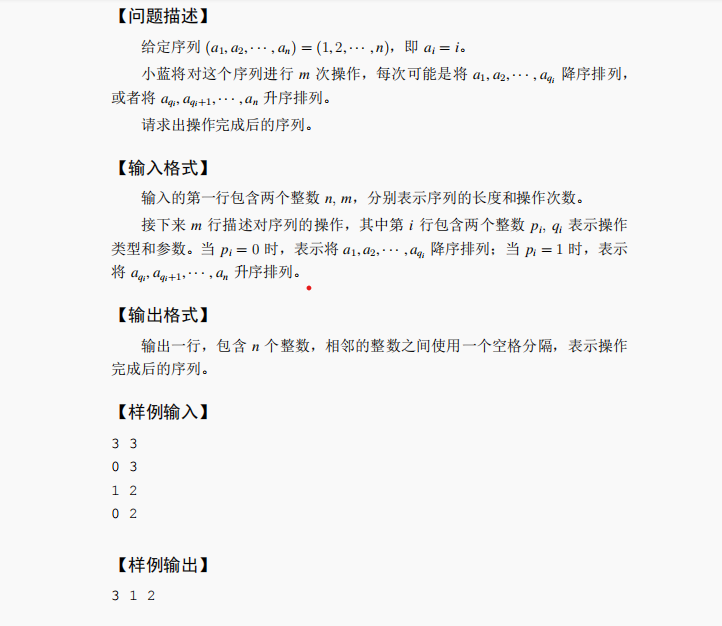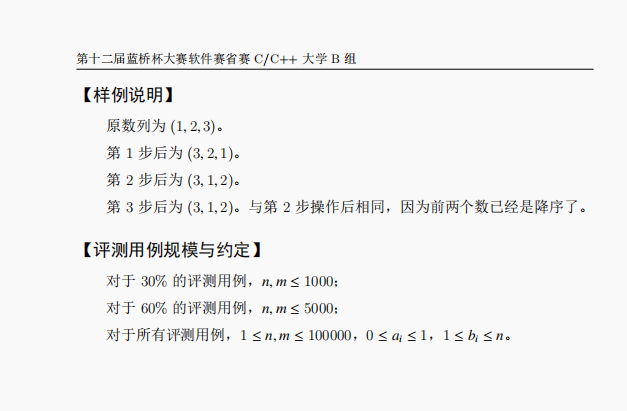这题主要考的就是升序降序的应用，值得注意的一点是下标控制 下标一定要减一！！！我踩了不少坑 就是 我就是API调用师 哈哈哈

import java.util.Arrays;
import java.util.Scanner;
public class 双向排序 {
public static void main(String[] args) {
Scanner scanner = new Scanner(System.in);
int n = scanner.nextInt();
int m = scanner.nextInt();

int a[] = new int[n];//创建数组

//给数组赋值   1到n
for(int i = 0 ; i < n ; i ++){
a[i] = i + 1;
}

//m次操作
for (int i = 0; i < m; i++) {
int control = scanner.nextInt();
int index = scanner.nextInt();

if (control == 0) {
//调用接口sort先升序在反序等于逆序
Arrays.sort(a,0,index);

for(int x = 0 , y = index -1; x < y ; x ++ , y --){
int temp = a[x];
a[x] = a[y];
a[y] = temp;
}

}else if(control == 1){
//注意的一点  这里要减一才是数组的起始下标  将index-1到数组结尾进行升序
Arrays.sort(a,index - 1,n);
}
}
//输出数组
for (int a1:a) {
System.out.print(a1+" ");
}
}
}


展开全文• 第十二届蓝桥杯省赛JAVA B组双向排序个人题解 欢迎交流与纠正。 import java.util.Scanner; public class Main { public static void fac(int a,int b,int ans1[]) { int s=0; if(a==0) { for(int i=0;i...
第十二届蓝桥杯省赛JAVA B组双向排序个人题解
欢迎交流与纠正。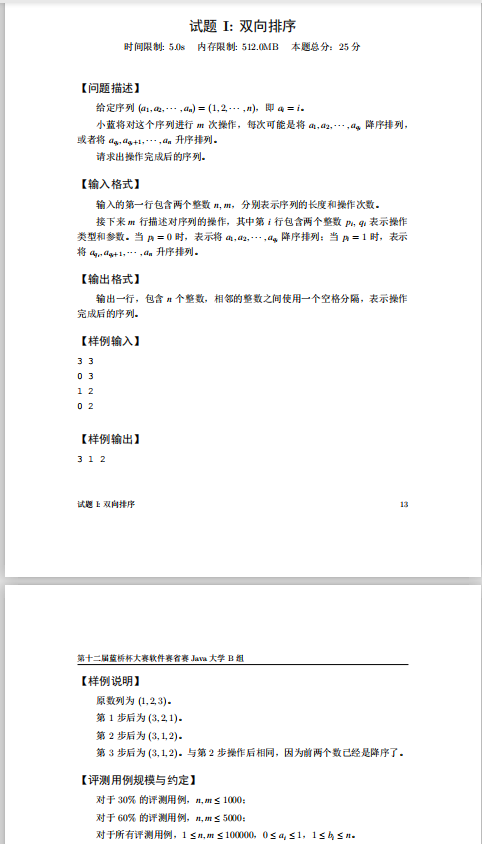import java.util.Scanner;
public class Main {
public static void fac(int a,int b,int ans1[])
{
int s=0;
if(a==0)
{
for(int i=0;i<b-1;i++)
for(int j=0;j<b-i-1;j++)
{
if(ans1[j]<ans1[j+1])
{
s=ans1[j];
ans1[j]=ans1[j+1];
ans1[j+1]=s;
}
}
}
if(a==1)
{
for(int i=0;i<ans1.length-b;i++)
for(int j=b-1;j<=ans1.length-b-i;j++)
{
if(ans1[j]>ans1[j+1])
{
s=ans1[j];
ans1[j]=ans1[j+1];
ans1[j+1]=s;
}
}
}
}
public static void main(String[] args) {
Scanner in=new Scanner(System.in);
int n=in.nextInt();
int m=in.nextInt();

int ans[]=new int[n];
for(int j=1;j<=n;j++)
{

ans[j-1]=j;
}
for(int i=0;i<m;i++)
{
int x=in.nextInt();
int y=in.nextInt();

fac(x,y,ans);

}

for(int o=0;o<ans.length;o++)
System.out.print(ans[o]+" ");
}
}

展开全文• 原题见本人上篇 本人萌新一枚欢迎指正。 import java.util.Arrays; import java.util.Collections; import java.util.Scanner; public class Main { public static void fac(int x,int y,int b[]) ...
• 1.1.1. 对区间[1,x][1,x][1,x] 进行降序排序 2.2.2. 对区间 [x,n][x,n][x,n] 进行升序排序 输出最后的数组aaa。 (1≤n,m≤100000)( 1 \leq n,m \leq 100000)(1≤n,m≤100000) 题解： sortsortsort只能拿一点分。根据...
• 行业分类-设备装置-基于隐空间学习和双向排序学习的跨媒体排序方法.zip
• 幼儿园教案2021-幼儿园中班数学活动：双向排序.doc
• 用C语言开发的双向冒泡排序算法，具体内容详见代码。
• 主要介绍了浅析java双向冒泡排序算法,并附上源码，需要的朋友可以参考下
• 冒泡排序算法应该算是每个开发者入门必学的基础算法，它逻辑清晰简单，代码实现也并不复杂，这里用自己的话语来总结一下。 （这些为了方便随便打开了一个node项目写的，其他Java ，PHP，C等也是一样的道理，算法讲究...算法 java node.js
• 1 #include 2 3 using namespace std; 4 #define swap(a,b) {int t;t = a;a = b;b = t;} 5 //节点类型的定义 6 typedef struct node 7 { 8 int data;...//双向冒泡 96 Traverse(L);//遍历链表 97 return 0; 98 }
•  冒泡排序算法的运作如下：（从后往前）  1.比较相邻的元素。如果第一个比第二个大，就交换他们两个。  2.对每一对相邻元素作同样的工作，从开始第一对到结尾的最后一对。在这一点，最后的元素应该会是最大的数...C++
• 因此针对这一问题，产生了双向冒泡排序法，也称鸡尾酒排序法。 双向冒泡排序法： 由两个方向同时进行冒泡： 首先由左向右为大元素移动方向，从右向左为小元素移动方向，然后每个元素都依次执行。 在第i次移动后，...
• ## 双向链表排序

万次阅读 2018-07-17 20:52:18
• 双向冒泡排序算法-Java实现 算法思想： 类似于冒泡排序 首先是定义两个边界即left和right 其次通过left右移进行冒泡排序，即将最左边数据与之后数据的不断比较交换，直到第一个数据为最小，之后left++； right的操作...java
• C++双向链表排序 建立一个长度为n的带头结点的双向链表，使得该链表中的数据元素递增有序排列。（必须使用双向链表完成，数据类型为整型。） 输入 第一行：双向表的长度； 第二行：链表中的数据元素。 输出 ...C 数据结构
• 前几章讲了交换排序中较为复杂切高效的堆排序，这次就来讲讲选择排序中最简单的直接选择排序及其改进双向选择排序。 选择排序：顾名思义，就是主要通过选择来完成的排序，每一趟从待排数据中选择一个最大或者最小的...算法 数据结构 c语言
• delphi listview可以双向排序，直接运行
• 主要为大家详细介绍了C++实现双向冒泡排序算法，文中示例代码介绍的非常详细，具有一定的参考价值，感兴趣的小伙伴们可以参考一下
• //计数排序 TypeName void Sort(T R[],int n) { int l = 0, r = n - 1; while (l) { for (int i = l; i ; i++)//由左向右扫描，将最大值置于右边 { if (R[i]>R[i + 1]) SWAP(R, i, i + 1); } --r;...
• NULL 博文链接：https://z-jls03.iteye.com/blog/836195
• 双向冒泡排序 首先从前往后两个两个比较把大的数移到最后一个位置，然后从后往前两个两个比较把小 的的数后往前推到数组第一个位置，这一过程就是第一轮这个时候第一个位置就是数组的 最小值，最后一个位置就是数组......